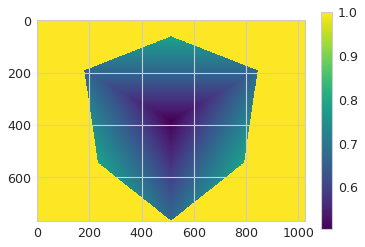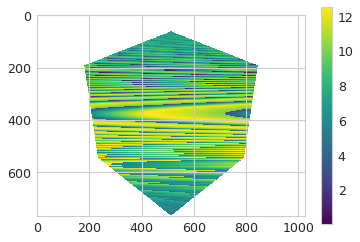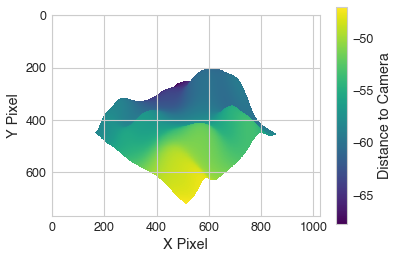# Absolute Values for Depth Image

When using the `vtk.vtkWindowToImageFilter` to produce a buffered image of the distances to the mesh in the rendering scene, is it possible to use the 0-1 depth ranges to recover the true distances from the camera to the mesh?

``````...
alg = vtk.vtkWindowToImageFilter()
alg.SetInput(ren_win)
alg.SetInputBufferTypeToZBuffer()
alg.Modified()
alg.Update()
image = alg.GetOutput()
...
``````

I image I can use these 0-1 depth values and the camera projection angles to recover the true distances but exactly how would I approach this?

0 is the near clipping plane, 1 is that far clipping plane. The in between values depend on if you are in parallel or perspective mode. For parallel it is just linear between near/far. For perspective

``````zbuff = 0.5 + (near + far + 2*near*far/zval)/(2*(far - near))

zbuff - 0.5 = (near + far + 2*near*far/zval)/(2*(far - near))

(zbuff - 0.5) *2*(far - near) = near + far + 2*near*far/zval

(zbuff - 0.5) *2*(far - near)  - near - far = 2*near*far/zval

((zbuff - 0.5) *2*(far - near)  - near - far)/(2*near*far) = 1/zval

2*near*far/((zbuff - 0.5) *2*(far - near)  - near - far) = zval
``````

Where zbuff is between 0 and 1.0, near and far are the near and far clipping distances (always positive) and zval is the distance on the view plane normal from the camera (always negative values with VTK right handed coord system)

1 Like

What about if the view is set to perspective? Is there any functionality in VTK to assist in these calculations?

Currently, I’ve gotten myself down a rabbit hole where I think I may be close to the desired result:

``````...# Got the image from the rendering scene as `img` NumPy image array
import numpy as np
import vtki
import matplotlib.pyplot as plt

plt.imshow(img)
plt.colorbar()
plt.show()
``````Then I get a point cloud:

``````def get_depth_pc(plotter,):
ren_source = vtk.vtkRendererSource()
ren_source.SetInput(plotter.renderer)
ren_source.WholeWindowOff()
ren_source.DepthValuesOn()
ren_source.Update()
pc = vtk.vtkDepthImageToPointCloud()
pc.SetCamera(plotter.renderer.GetActiveCamera())
pc.SetInputConnection(ren_source.GetOutputPort())
pc.Update()
return vtki.wrap(pc.GetOutput())

point_cloud = get_depth_pc(p)
``````

Then I transform the points to the camera’s reference frame

``````def get_numpy_mtx(mtx):
vals = np.empty((3,3))
for i in range(3):
for j in range(3):
vals[i,j] = mtx.GetElement(i, j)
return vals

matrix = p.camera.GetModelViewTransformMatrix()
mtx = get_numpy_mtx(matrix)
points = point_cloud.points.dot(mtx)
``````

Then I attempt to produce the depth mapped image… but it doesn’t look right:

``````idx = np.argwhere(img.ravel() != 1.0).ravel()
depths = np.empty_like(img.ravel())
depths[:] = np.nan
depths[idx] = points[:,-1]
plt.imshow(depths.reshape(img.shape))
plt.colorbar()
plt.show()
``````VTK also has

renderer->DisplayToWorld(x,y,z)
renderer->GetWorldPoint()

So I was looking back at this thread with a colleague as we’re implementing this depth mapping in PyVista as a part of a research project and we realized that I totally read your original post wrong… turns out you answered exactly what I was asking (only took ~6 months to realize it).

For anyone who needs an elegant solution, check out this using PyVista (which will be updated in PyVista before long such the the `image_depth` attribute returns the image with depth to the camera like shown below):

``````import numpy as np
import pyvista as pv
import matplotlib.pyplot as plt
from pyvista import examples

pv.close_all()
p = pv.Plotter()
p.show(auto_close=False)

near, far = p.camera.GetClippingRange()
zval = 2*near*far/((p.image_depth[:,:,0] - 0.5) *2*(far - near)  - near - far)
zval[zval <= -far] = np.nan

plt.imshow(zval)
plt.colorbar(label='Distance to Camera')
plt.xlabel('X Pixel')
plt.ylabel('Y Pixel')
``````How do you change the view using intrinsic/extrinsic camera parameters?

It seems that inside the function `get_image_depth()`

But I am still missing the transformation from intrinsic/extrinsic to vtk camera?

Ok, I have a working version for python and pyvista (VTK):

I still have some questions regarding the offline rendering (off_screen=true). My questions: